Basic Triangle Values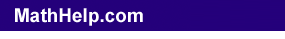There are a few (a very few) angles that have relatively "neat" trigonometric values, involving, at worst, one square root. Because of their relatively simple values, these are the angles which will typically be used in math problems (in calculus, especially), and you will be expected to have these angles' values memorized.

Usually, textbooks present these values in a table that you are expected memorize. But pictures are generally easier to recall (on tests, etc); this lesson will show the way in which many people really keep track of these values.

45°-Angle Values (from a 45-45-90 triangle)

 If you need to work with a 45-degree angle, then draw the 45-45-90-degree triangle at the right, using the given values for the lengths of the sides: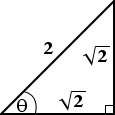We choose a length of one unit for the matching sides because this is simplest, and then we get the sqrt(2) value by using the Pythagorean Theorem. (Note that it is irrelevant what are the lengths of the actual triangle you are dealing with; this reference triangle gives you the ratios, and thus the trigonometric values.) The "theta" (the squashed circle with the line through it) in the lower left corner is the angle we'll use. (The orientation of the particular angle they want you to work with is irrelevant, because you can always rotate the above triangle to put it into whatever orientation you need.)

If, for a given exercise, they only want the trigonometric value of the angle, then just read it off the triangle: the sine of theta is (opposite) over (hypoteneuse), or 1/sqrt(2); the cosine of theta is (adjacent) over (hypoteneuse), or 1/sqrt(2); and the tangent of theta is (opposite) over (adjacent), or 1/1 = 1. (Your text or teacher might want you to "rationalize" these ratios, in which case you get sin(theta) = sqrt(2)/2 and cos(theta) = sqrt(2)/2. This rationalization issue becomes much less important once you reach calculus.)

On the other hand, suppose they've given you a 45-degree triangle where the two matching sides have length, say, 14 units, and they want you to find the length of the third side. Okay, you multiply 1 by 14 to get 14 for the matching sides. Then, by similar triangles, you multiply sqrt(2) by 14 to get 14sqrt(2) for the length of the hypoteneuse.

Or suppose they tell you that the hypoteneuse is 12 units. Let's figure out what we have to multiply by to get the lengths of the matching sides. Let this multiplier be "x". Then xsqrt(2) = 12, so x = 12/sqrt(2) = 12sqrt(2)/2 = 6sqrt(2). Then the lengths of the matching sides are 1×6sqrt(2)= 6sqrt(2) units.  Copyright © Elizabeth Stapel 2000-2011 All Rights Reserved

You can find anything you need from this reference triangle. Instead of trying to memorize an entire table (if that's not working for you), simply memorize this triangle.

Content Continues Below

30°- and 60°-Angle Values (from a 30-60-90 triangle)

If you need to work with a 30- or a 60-degree angle, the process is similar to the above, but the set-up is a bit longer.

 For either of the angles, this is the triangle that you start with:This is a 60-60-60 triangle (that is, an equilateral triangle), with sides having a length of two units. Drop the vertical bisector from the top angle down to the bottom side: Note that this bisector is also the altitude (height) of the triangle.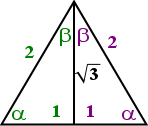By using the Pythagorean Theorem, we get that the length of the bisector is sqrt(3), and the bisector has formed 30-60-90 triangles. If you are doing a 60-degree triangle, use the angle labelled above as "alpha" (the funny-looking "a" in the lower corner); if you are doing a 30-degree triangle, use the angle labelled with "beta" (the funny-looking "b" in the upper corner). For working with 60-degree angles, your picture is this half of the triangle: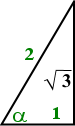...and for 30-degree angles, your picture is the same half, but rotated: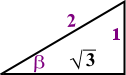You find trigonometric values and ratios with the 30-degree and 60-degree triangles in the exact same manner as with the 45-degree triangle.

You may get one of those teachers who doesn't want you to draw these pictures (because you're supposed to have everything memorized by now). Well, this is why your pencil has an eraser. My Calculus II instructor said that if we drew the pictures on our tests, the entire problem would be counted wrong. I drew the pictures anyway, but very lightly, and erased them all before I handed the tests in. He never knew, and I passed the course. You do what you gotta do.

On the other hand, some people prefer tables and charts. If tables work better for you, then this table comes highly recommended, having been "field-tested" by a working instructor:

 30° 45° 60° sin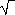1 2 3 cos3 2 1 divided by 2

To find, say, the sine of forty-five degrees, you would trace across in the "sin" row and down the "45°" column, taking the square-root symbol with you as you go and remembering to include the "divided by 2" from the bottom, to get sin(45°) = sqrt(2)/2. The neat pattern of "1, 2, 3" across the top row and "3, 2, 1" across the middle row are meant to help you memorize the table values. Keep in mind that the square root of 1 is just 1, so, for instance, cos(60°) = sqrt(1)/2 = 1/2.

 Cite this article as: Stapel, Elizabeth. "Basic Triangle Values." Purplemath. Available from     https://www.purplemath.com/modules/trig.htm. Accessed [Date] [Month] 2016

MathHelp.com Courses

Purplemath:

Study Skills Survey